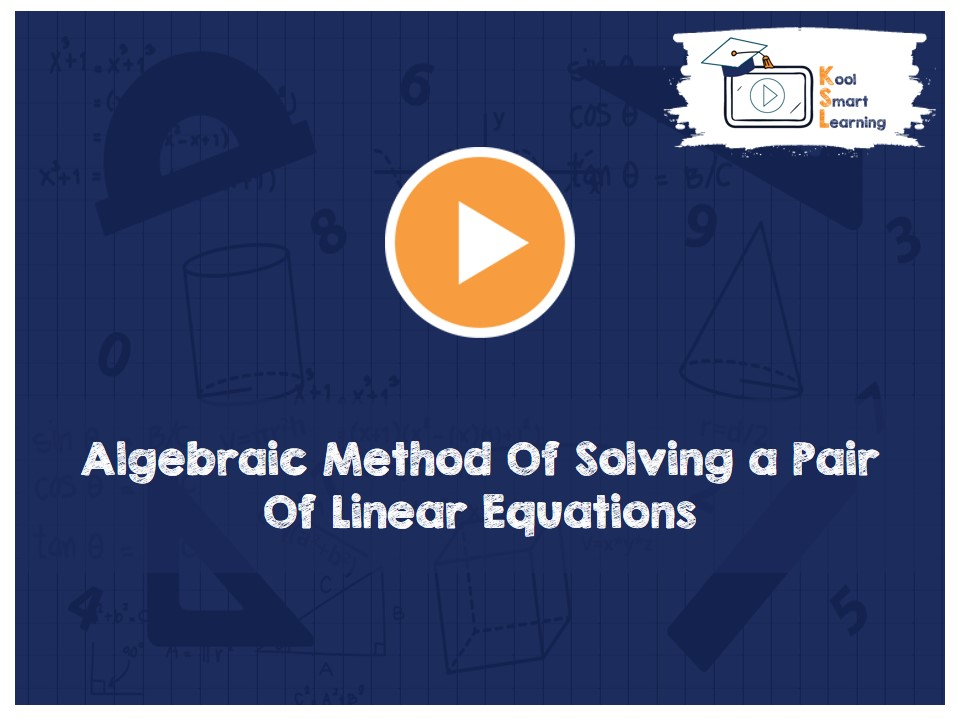At KoolSmartLearning, we intend to harness the power of online education to make learning easy.## Algebraic Method Of Solving a Pair Of Linear Equations

/  Algebraic Method Of Solving a Pair Of Linear Equations## Algebraic Method Of Solving a Pair Of Linear Equations

The video discusses the three methods of solving a Pair of Linear Equations in Two Variables algebraically which includes substitution method, elimination method & cross-multiplication method. The video explains how we derive the cross multiplication formula & also covers how these methods can be used to solve equations which are not linear equations.

More Related Videos